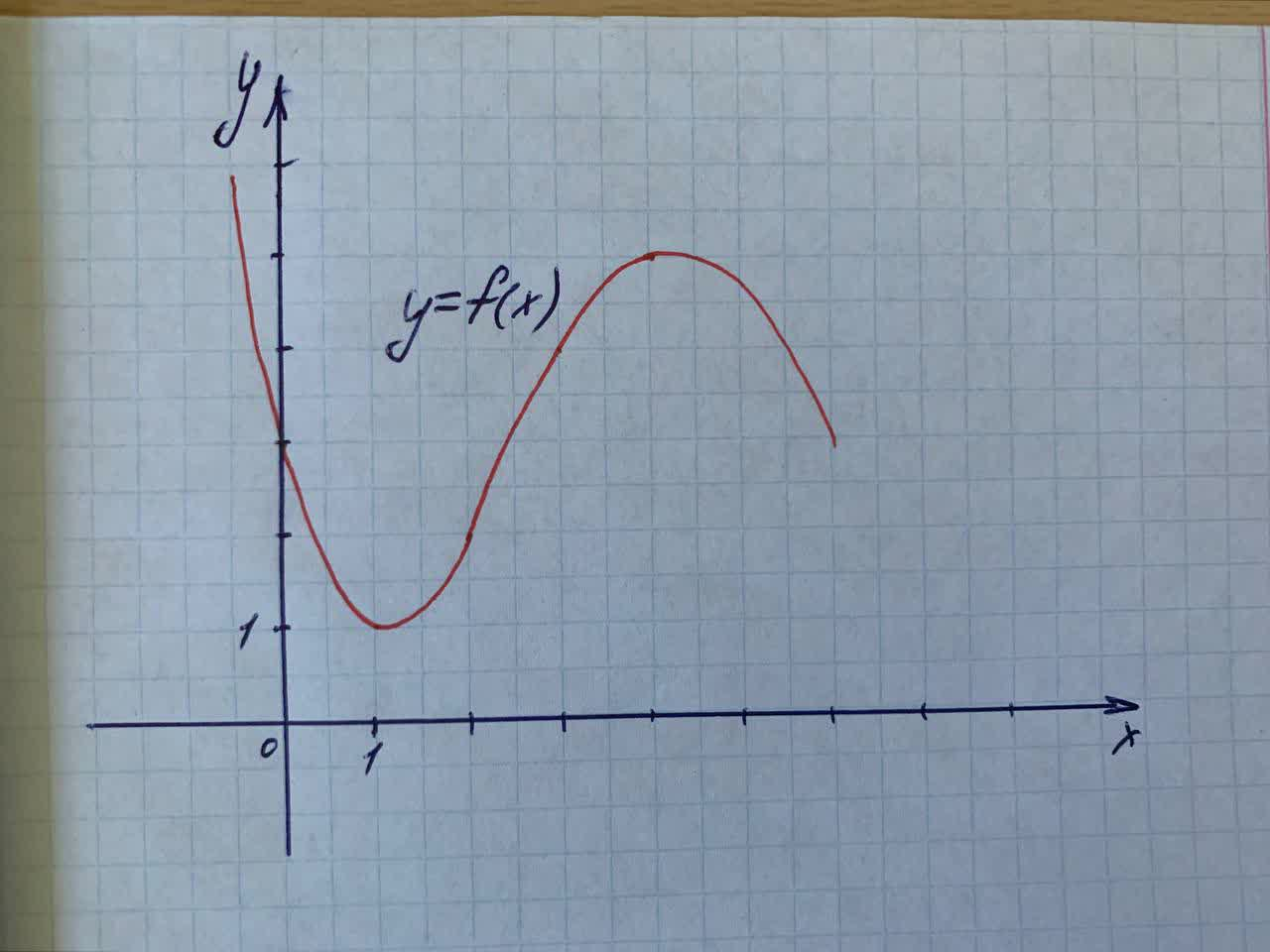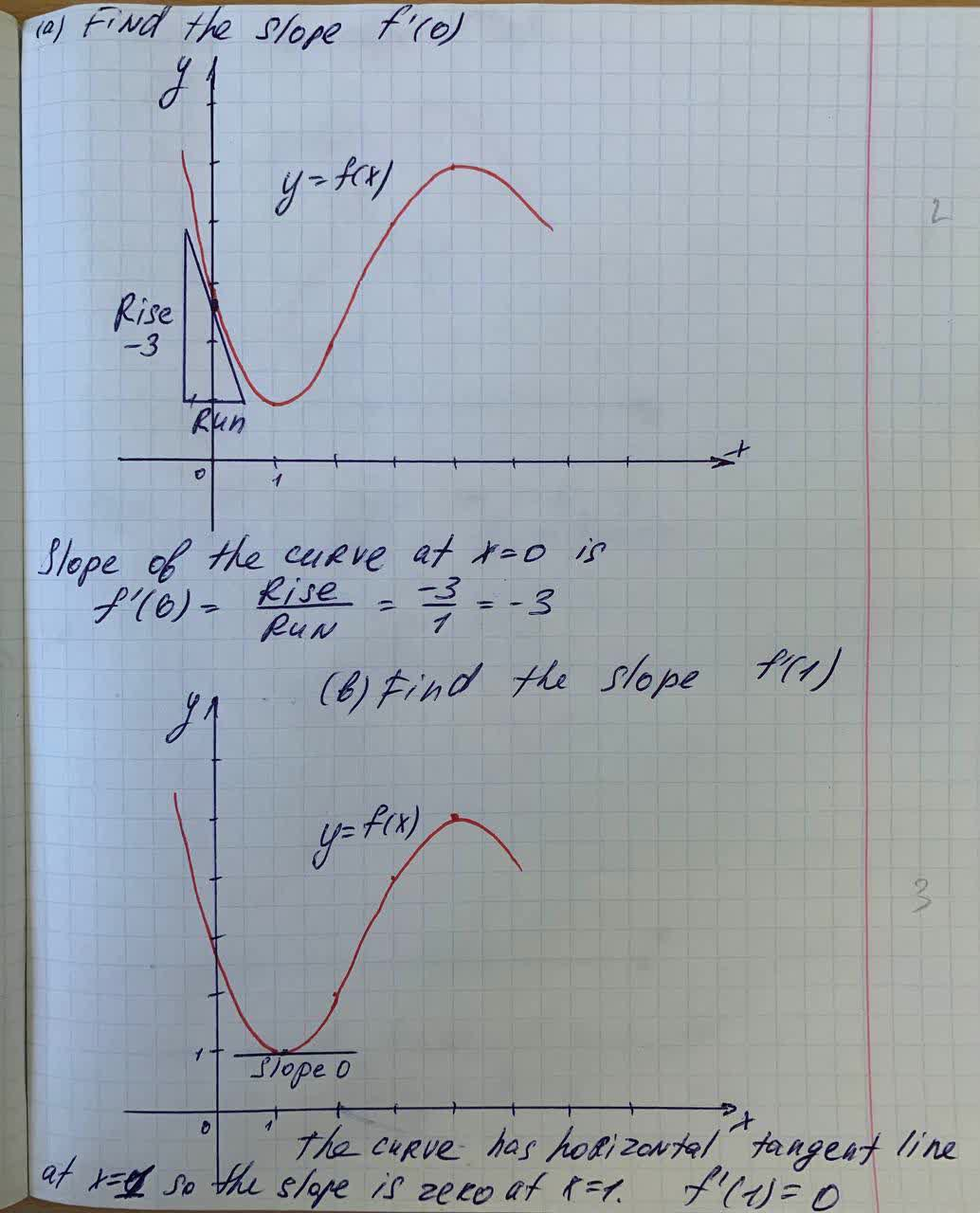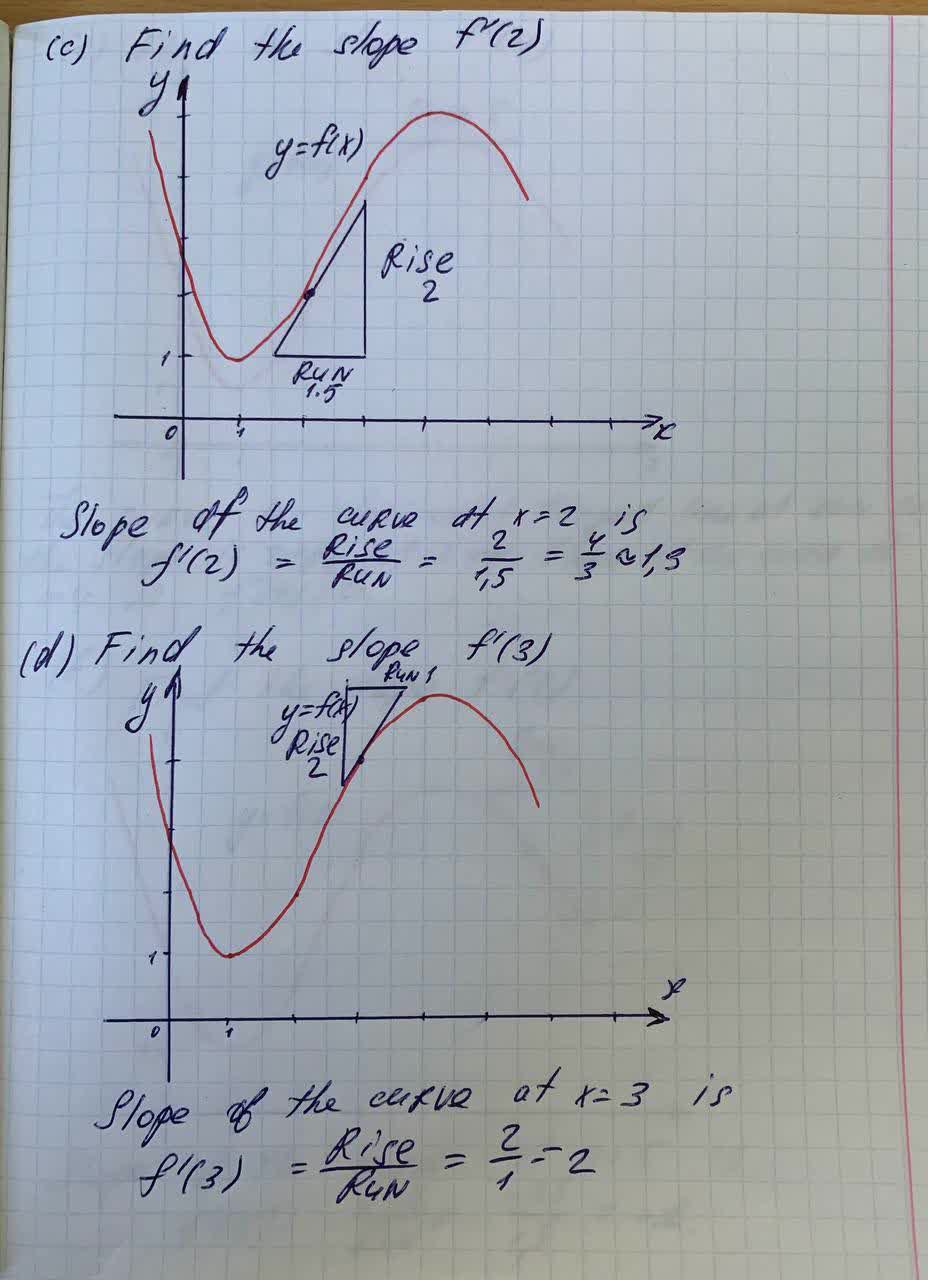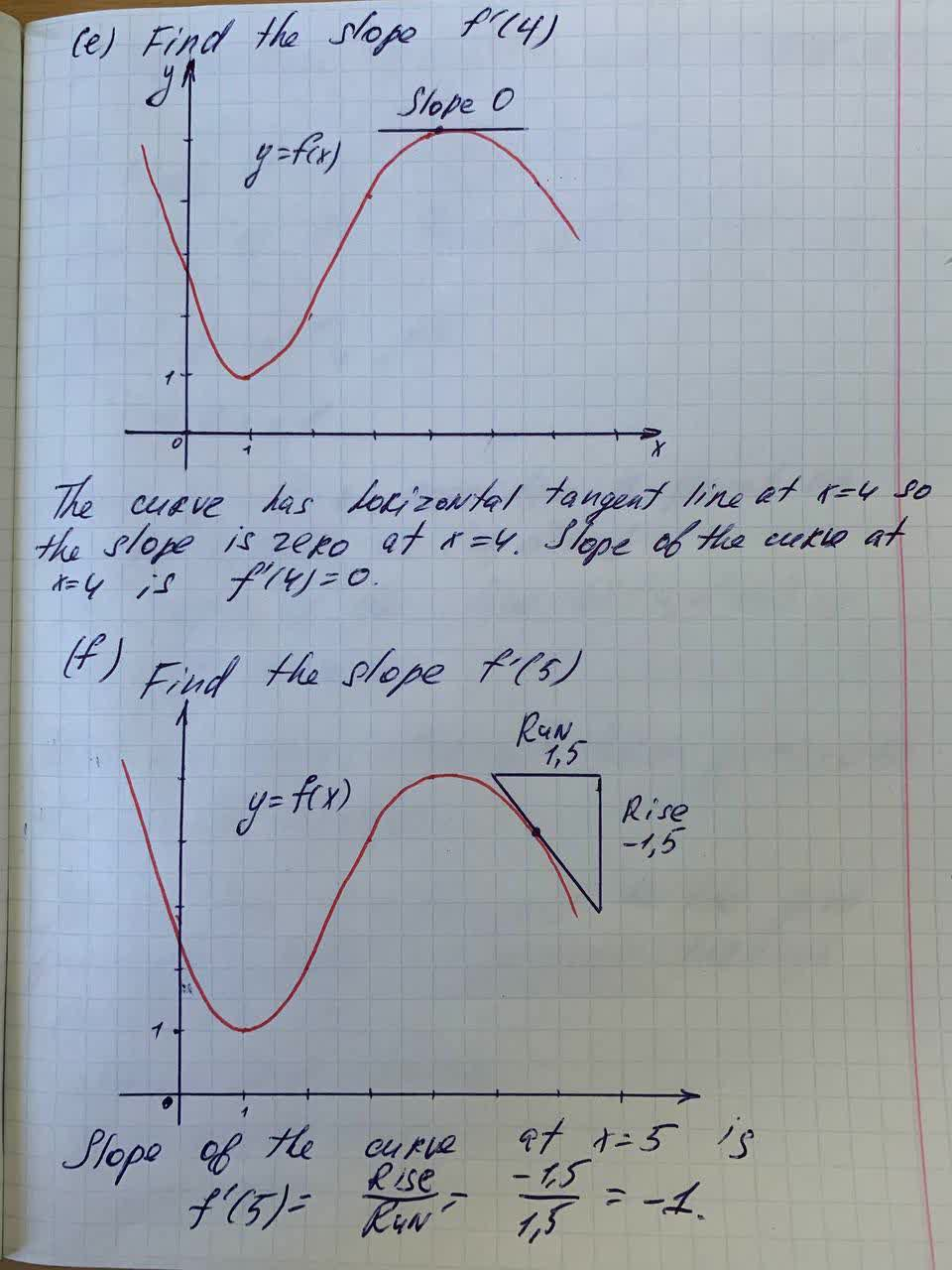Question# Use the given graph to estimate the value of each derivative.(Round all answers to one decimal place.) Graph uploaded below. (a) f ' (0) 1 (b) f ' (1) 2 (c) f ' (2) 3 (d) f ' (3) 4 (e) f ' (4) 5 (f) f ' (5) 6 12110500681.jpg

Derivatives
ANSWEREDUse the given graph to estimate the value of each derivative.(Round all answers to one decimal place.) Graph uploaded below.
(a) f ' (0) 1
(b) f ' (1) 2
(c) f ' (2) 3
(d) f ' (3) 4
(e) f ' (4) 5
(f) f ' (5) 6